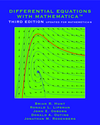Home
Project Description
Authors

Differential Equations
with Mathematica

Differential Equations
with Maple

Differential Equations
with Matlab

# Differential Equations with Mathematica, Third Edition

## Sample Problem

### Problem Set D. Second Order Equations

#### Problem 1.

Airy's equation is the linear second order homogeneous equation y" = ty. Although it arises in a number of applications, including quantum mechanics, optics and waves, it cannot be solved exactly by the standard symbolic methods. In order to analyze the solution curves, let us reason as follows.

(a) For t close to zero, the equation resembles y"=0, which has general solution y = c1t + c2. We refer to this as a "facsimile" solution. Graph a numerical solution to Airy's equation with initial conditions y(0) = 0, y'(0) = 1, and the facsimile solution (with the same initial data) on the interval (-2, 2). How well do they match?

(b) For t ≈ −K2 << 0, the equation resembles y" = −K2y, and the corresponding facsimile solution is given by y = c1 sin(Kt + c2). Again using the initial conditions y(0) = 0, y'(0) = 1, plot a numerical solution of Airy's equation over the interval (-18, -14). Using the value K = 4, try to find values of c1 and c2 so that the facsimile solution matches well with the actual solution. Why shouldn't we expect the initial conditions for Airy's equation to be the appropriate initial conditions for the facsimile solution?

(c) For tK2 >> 0, Airy's equation resembles y" = K2y, which has solution y = c1 sinh(Kt + c2). Plot a numerical solution of Airy's equation together with a facsimile solution (with K = 4) on the interval (14, 18). In analogy with part (b), you have to choose values for c1 and c2 in the facsimile solution.

(d) Plot the numerical solution of Airy's equation on the interval (-20, 2). What does the graph suggest about the frequency and amplitude of oscillations as t → -∞? Could any of that information have been predicted from the facsimile analysis?

Here is a Mathematica Notebook solution, converted to a PDF file. If your browser is configured to view Mathematica Notebooks, you can view the actual Mathematica Notebook solution.